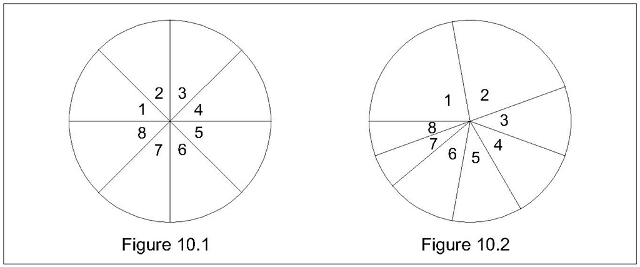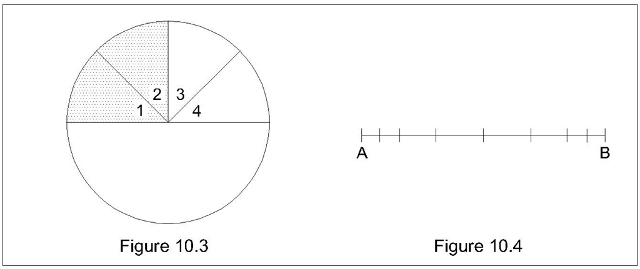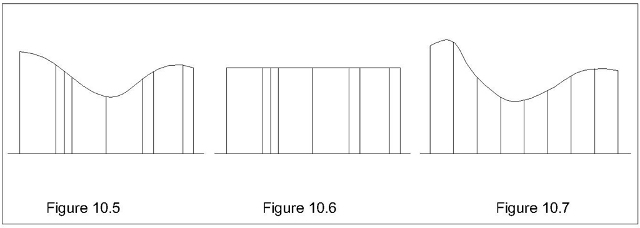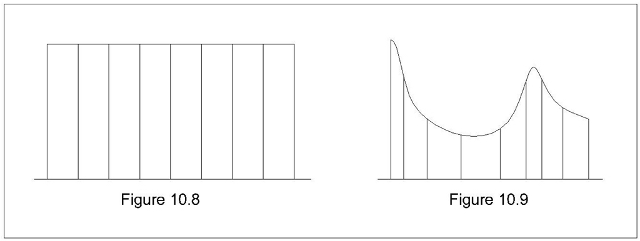CHAPTER 10

ASSIGNATION

10.1. In this chapter I face the problem concerning the assignation of the respective measures to the alternatives partitioning a possibility space (assignation problem).

Even once agreed that henceforth sortal trivalence is abandoned, so that the third alethic value depends exclusively on a lack of information about proper propositions, and even once agreed that such a lack of information depends exclusively on the institutive stage (§9.2.1), a momentous ambiguity survives because, so to say, a statute can be affected by at least two kinds of informational lacks entailing different consequences on the undecidability of the hypothesis h to collate. In fact sometimes h is probabilistically valuable and sometimes it is not. In other words, while it is reasonable to classify all the dilemmas whose two horns are true and false as (alethically) decidable, to classify as undecidable all the dilemmas which are not decidable entails (at least) a missing distinction between probabilistically valuable and probabilistically unvaluable dilemmas.

Let me enter into details. For the sake of simplicity I will mainly reason through ® on Ω°.

10.2. In ® the assignation problem is the problem of quantifying the areas of the sectors involved by such fields, that is the problem of establishing the number and the position of the radii separating the various sectors. For instance, with reference to the aforementioned slider on the rail, if the assignation is uniform (k1 assigns the same measure to the eight alternatives) we obtain Figure 10.1 (reproducing Figure 6.1)exactly as we obtain Figure 10.2 (reproducing Figure 6.2) if the measure k2 assigns to alternatives 1 and 2 is the double of the measure assigned to alternatives 3, 4, 5, 6 and the quadruple of the measure assigned to alternatives 7 and 8. Anyhow a statute may also concern only a part of the tract. For instance, while the statute k3 represented in Figure 10.3tells us that the first half of the tract is uniformly tetra-partitioned and that the slider is not in its first quarter, it does not tell us anything about the second half of the same tract. Under this k3, while h1

(10.i)       the slider is in segment 1

is a decidable (and false) hypothesis, both h2

(10.ii)       the slider is in segment 3

and h3

(10.iii)       the slider is in segment 5

are undecidable. Yet a momentous difference exists between (10.ii) and (10.iii): in fact we can assign a probabilistic value (1/8, obviously) to the former, but not to the latter. The probability (under k) of a h is the ratio between the k-measure of h and the k-measure of the same k, therefore it is represented by the ratio between the respective virgin fields. So the impossibility to draw the second radium delimitating sector 5 (whose positions we do not know) forbids the identification of the respective measure. An even more undecidable hypothesis is

(10.iv) the slider is in segment 7

for the same existence of a segment 7 is unknown.

10.3. In this sense a refinement of the classification proposed in §8.4 is needed. In accordance with it, a h-incomplete statute is either h-adequate (if it allows the assignation of P(h|k)) or h-defective. Reciprocally a k-undecidable hypothesis h is either k-valuable (for instance (10.ii)) or k-unvaluable (for instance (10.iii)).

A statute is absolutely adequate iff it is h-adequate for every h concerning its possibility space; an absolutely adequate statute is represented by a totally partitioned circle. Reciprocally a circle whose partition is totally unknown represents an absolutely defective (or empty) statute. For instance Figure 10.1 and 10.2 represent two absolutely adequate statutes. Instead the statute k3 represented in Figure 10.3 is h1-exhaustive, h2-adequate, and h3-defective, so showing that the same statute may be exhaustive, adequate and defective in dependence of the hypothesis under scrutiny.

Of course an absolutely exhaustive statute is represented by a circle whose sectors but one are shaded, that is, under the re-partitioning technique, by a circle which is also its only sector.

10.4. For the sake of pedantry I remark that the same notion of h-adequateness could be ulteriorly refined by the distinction opposing strong and weak h-adequate statutes. Let me sketch such an opposition through an extremely simple pair of connected examples.

Example I. Under the following statute k1

a)      in an urn m there are two counters (m=m2)

b)       one counter is blue and one is red ((m=m1+1 )

c)       the other physical characteristics of the counters (size, material, shape et cetera) are exactly equal

d)      the withdrawing mechanism is chromatically impartial (no privileging photoelectric apparatus)

the theoretic probability of withdrawing a blue counter is

P(B| k1) = 1/2

and the frequency we can empirically realize is 1/2 too. In order to express this identity we could say that k1 is strongly adequate as for the mentioned hypothesis.

Example II. A third equal counter is inserted (blue? red? We only know that no colour is privileged). Under this new statute k2 the theoretic probability of withdrawing a blue counter is

P(B|k2) = 2/3(1/2)+1/3(1/2)

therefore it is again 1/2. Yet the limit value of the frequency we can empirically realize will be either 2/3 (if m=m2+1) or 1/3 (if m=m1+2) surely not 1/2. In order to express this non-identity between theoretic probability and frequency we could say that k2 is weakly adequate as for the mentioned hypothesis II.

I do not dwell on this rather marginal topic. Simply I remark that the reason of the discrepancy is that the Example II refers to a second order probabilistic problem, that is to a context where the probabilistic value is grounded on data which are in their turn grounded on probabilistic data. This means that in ® the weakly adequate (the second order) partition of the circle does not correspond to any composition of the urn; in fact, since we ignore its real composition, so to say, such a second order partition results from a pondered valuation of the two first order partitions (I mean (m=m2+1) ↓ (m=m1+2)), each of them representing a strongly adequate statute (no doubt that if we compose 109 urns under k2, the number of m2+1 tends to 109/2).

Henceforth I will only speak of adequate statutes, leaving out of consideration the distinction between strong and weak adequateness.

10.5. The considerations above do not involve the theme concerning any eventual subjective intervention in the assumption of a statute Here I tackle it.

Once fixed the hypothesis h under scrutiny, the promotion of an h-defective statute to h-adequateness or of an h-adequate statute to h-exhaustiveness is realized by acquiring new pieces of information improving the same statute. Since the procedure is the same, for the sake of concision I will only treat the former case.

Actually in every moment of our life we need to take decisions about undecidable hypotheses; in fact they concern possibility spaces whose complexity is too high to be analytically framed and whose context does not allow the acquirement of sure data able to promote the previously defective statute we are dealing with. Therefore, in order to take such decisions, we must resort to some vague and personal estimates. A classical example is the bookmaker who rates the next match; of course such rates are inversely proportional to his opinion about the respective chances, but evidently any mathematically quantifiable (therefore objective) analysis of the thousand factors influencing these chances (influencing this assignation of measures) is beyond his cognitive possibilities. In this sense I speak of a subjective intervention. Yet the subjectivism I am speaking of, in opposition to the fundamentalist subjectivism in the style of de Finetti, is a critical and integrative subjectivism. It is critical because the subjective intervention is not arbitrary in the broad sense that everyone is free to assign the measures he prefers; these measures have to comply with a sensible estimate of the situation, and such an estimate must be strictly inferred from the contingent physical context of the phenomenon under scrutiny (bookmakers who were to pay a high rate for a nearly sure winner would be condemned to a prompt extinction). It is integrative because, though critical, the subjective intervention is legitimate exclusively where an insufficient knowledge of the same context makes a personal assignation the only reasonable alternative to a sterile suspension of any judgement (the classical epoch). Even a scale of subjectivity could be proposed in compliance with the ratio between objective and subjective components in the statute on whose basis the assignation is achieved.

Two comments are opportune.

10.5.1. Under my severely deterministic approach (but are there deterministic approaches which are not severely deterministic?) *probability* and *ideal knower* are almost incompatible notions, because they lead to an apparent contradiction. On the one hand, for an ig whatever hypothesis is either true or false, therefore there is no room for any properly probabilistic approach. On the other hand, for instance, stating that, when we toss a (well balanced) die, the probability of a certain result is 1/6 is stating an exact and unobjectionable truth.

No contradiction: ig knows the next result because he (she?), knowing the specific values of the parametric quantities, knows such a specific rototranslatory run of the die et cetera; but if we make reference to a generic toss we leave institutionally out of consideration the specific parameters characterizing any specific toss, therefore we introduce, so to say, an institutional lack of information subjecting even ig. In this sense the range of probabilistic values has an absolutely objective import.

In other words. The value of 1/6 expresses the unobjectionable fact that 1/6 is exactly the value a correctly performed frequency (henceforth, a reliable frequency or even, shortly, a frequency) tends to.

10.5.2. Carnap (and followers) claim that the frequency is a second kind of probability. In my opinion a frequency is simply the empirical way to reach a certain result, exactly as the time necessary to fill this tub by this water-tap can be experimentally ascertained even when the strange shape of the tub or the sobbing flow from the water-tap renders very hard any mathematic computation. Analogously, where both a theoretical assignation and a reliable frequency are realizable, the latter can control the former (either validating or invalidating it), and where no theoretical assignation is possible, the reliable frequency supplies an otherwise unachievable datum.

Of course an invalidating reliable frequency (a discrepancy between empirical and theoretical assignations), once excluded a too hasty conclusion suggested by some strange contingent peculiarity (as for instance the famous 21 consecutive rouge in Montecarlo) is an unobjectionable symptom of some mistake in the theoretical approach, that is of some mistake affecting the objective computation or (inclusive) the subjective intervention; thus the non-arbitrariness of the same subjective intervention is argued.

10.6. My basic claim is that the assignation of a measure to the various alternatives concerning a possibility space (that is a partition of the circle) is dictated by our knowledge of the physical phenomenon the same possibility space refers to. In order to argue about this claim I enter into details of Ω°; that is I analyse the procedure allowing me to assign a respective measure to the eight possible alternatives (from now on "upshot", symbolically "u", is the technical term to mean the result of a throwing). Given a certain impulse (a certain value of the parametric quantity I) and a statute specifying the various resistances to the motion (frictional, gravitational, aerodynamic, magnetic and so on) that the slider meets from point to point (let R be their resultant), rational mechanics establishes where the slider will stop (that is the value of the covered distance L such that I is the integral of RdL from 0 to L). In order to avoid superfluous complications here too (§6.11) I assume that R is the same in every point of the tract, so that a direct proportionality exists between covered distances and impulses (let me insist: this simplification is not at all a theoretically reductive trick to introduce implicitly a positional equiprobability). Thanks to such an R-uniformity the octo-partitioned segment AB of Figure 10.4 can be indifferently interpreted as the iconic representation of the tract or as the (non-iconic) representation of DI that is of the impulsive range Imax- Imin. (where Imin and Imax are the impulses necessary to reach respectively the initial point of section 1 and the final point of section 8).

10.6.1. The probabilistic character of the problem depends on the fact that I is a parametric quantity. Of course the links between *parametric quantity* and *casual variable* are many, yet I follow my way without dwelling on a rather secondary theme; therefore I say that Ω° is a possibility space ruled by the parametric quantity I over the range DI (only impulses belonging to DI will be considered).

Let me recall (ibidem) the flexibility of the example. Contrary to a die, where, say, an epta-partition of the possibility space would be rather difficult, here it would be easy to replace the octo-partition with an epta-partition, for instance by annexing section 2 to section 3 or by re-partitioning the entire tract. The crucial passage is that, since the potential final positions of the slider are ‘infinite', any finite partition (for instance our octo-partition) entails that physically different upshots must be considered equivalent as for their probabilistic classification.

So, for instance, let I4min and I4max be the minimal and maximal impulses relative to the section 4; I call "parametric differential (relative to the section 4)" the value D4I=I4max-I4min. Generically but propaedeutically speaking the parametric differential Dj(x) relative to a certain parameter X and to a certain upshot uj is given by all the parametric values determining uj. In the next chapter more punctual considerations will be proposed.

10.6.2. To object that both *parameter* and *parametric differential* are marginal notions because we can speak of probability also with reference to single events, seems to me a naivety. In fact the assignation of a probability to a single event is only possible if we insert the single event in a parametric context. For an omniscient subject the probability p that I will be alive in ten years is anyhow a certainty: either negative or (as I am inclined to hope) positive; in fact his omniscience allows him to know the future of that single and incomparable (in the most immodest acceptation) individual I am. But the probabilistic result of any non-omniscient approach will depend on the set I am inserted in: the probability p1 relative to my being a seventy-years old Italian without severe diseases is greater than the probability p2 relative to my being a person whose mother and maternal grand-father died at fifty by ictus; et cetera. And even if we claim that p is the pondered average of the various pi, we cannot avoid the conclusion that the probability of a single event passes necessarily through parametric probabilities.

10.7. Coming back to our slider, concluding that the measure of each alternative (therefore the areas of the eight sectors occurring in a ®-diagram) must be directly proportional to the respective parametric differentials (therefore to the lengths of the respective segments occurring in Figure 10.4) would be a serious mistake. In fact concluding that the probability of a generic upshot uj is given by the ratio Dj(I)/ D(I) is postulating implicitly the uniform distribution of the impulses over the whole range D(I) because, evidently, if the rule determining the charge of the spring privileges some values, the corresponding upshots too are privileged. In other words, such a postulation is abusive, since a uniform distribution of the impulses is a possibility, not a necessity.

10.7.1. I call "density function" (symbolically "y(I)") the function establishing how the various I-values are distributed over D(I). So the measure assigned to the j-alternative is directly proportional to

(10.v) Dj(I)yj(I)

where yj(I) is the average value of the density function on the respective differential (strictly, (10.v) ought to be replaced by the integral of the y–function from Ij,min to Ij,max).

The coefficient of proportionality between measures and (10.v) depends on the choice of a unit, but it is of no theoretical moment (§7.15); for instance, with reference to Figure 10.5(where a generic density function is represented in ordinate), the important factor is the ratio between the area of any single mixtilinear rectangle and the area of the whole figure, and such a ratio, manifestly, is not at all influenced by the unit we choose.

10.7.2. Therefore, shortly,

(10.vi)       P(uj|k) = Dj (I)yj(I) / Σ(Dj(I)yj(I))

is the general formula, and

(10.vii)       P(uj|k) = Dj (I) / Σ(Dj (I))

is the particular formula where the uniformity of the density function allows the respective simplification.

10.7.2.1. From a formal viewpoint it must be remarked that the reason why "k" does not occur in the second members of (10.vi) and (10.vii) is that the parametric differential and the density function can only be determined through a statute; however, if we prefer a heavier but more explicit formulation "Dj" and "yj" can be replaced by "Dj k" and "yj,k" respectively.

10.8. Two particular cases are represented in Figure 10.6 and 10.7. In the former the density is uniform (therefore the areas are directly proportional to the parametric differentials (to the bases of the rectangles). In the latter the partition in parametric differentials is uniform, therefore the areas are directly proportional to the density (to the average heights of the rectangles). A doubly particular case is represented in Figure 10.8,where both the differentials and the density are uniform, and consequently the upshots are equiprobable (the various rectangles have the same area).

Equiprobable upshots (rectangles of the same area) are also obtained where (Figure 10-9) both parametric differentials and densities are non-uniform, but an inverse proportionality exists between them, so that, according to (10.v), their product is invariant. These two kinds of equiprobability can be distinguished by calling "uniform" the former and "compensative" the latter.

10.9. The proposed analysis shows that an assignation, besides depending on the parametric partition, depends on the density function y too. Then an easy puzzle (whose solution will be exposed in the next chapter) runs as follows. In the usual games of chances only the parametric partition is ascertained; for instance we see that every number has a sector of equal width in the rotating disk of a roulette, but we do not know the distribution of impulses transmitted by the croupier to the same disk and to the rolling ball: under the obvious condition that the roulette is well balanced, how on earth is the equiprobability of the upshots universally accepted? In other words: how on earth can we presuppose the uniformity of a density function we do not know? Particularly because this universally accepted presupposition is not limited to equiprobable assignations. For instance if we roll two dice, the partition is heteroprobable (1/36 for a two or a twelve, 1/18 for a three or an eleven et cetera until 1/6 for a seven), but this heteroprobability respects exactly the combinatory mathematics and consequently, in the end, the partition of parametric differentials; therefore Figure 10.6 tells us that here too a uniform y is postulated.